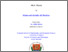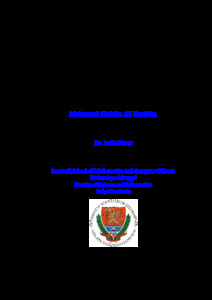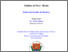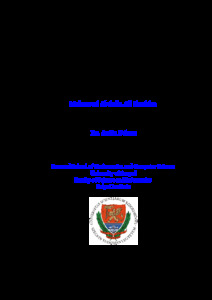# Threshold dynamics in mathematical models for mosquito- and rodent-borne diseases with seasonality

Ibrahim Mahmoud Abdalla Ali
Threshold dynamics in mathematical models for mosquito- and rodent-borne diseases with seasonality.
[Thesis]Preview PDF (thesis) Download (13MB) | PreviewPreview PDF (booklet) Download (1MB) | Preview

## Abstract in foreign language

The Ph.D. thesis investigates the impact of the periodicity of weather on the spread of malaria, Zika fever, and Lassa fever by applying non-autonomous compartmental population models with time-dependent (periodic) parameters. The dynamics of the system is characterized by the basic reproduction number ($\mathcal{R}_0$) of periodic compartmental models, defined as the spectral radius of an integral operator acting on the space of continuous periodic functions, and it has also been shown that the reproduction number is a threshold parameter with respect to disease extinction or persistence. Our aim is to show that the disease-free periodic solution of our newly established models is globally asymptotically stable if $\mathcal{R}_0 < 1$, while for $\mathcal{R}_0 > 1$, there exists at least one positive $\omega$-periodic solution. We provide numerical studies and give examples to describe what kind of parameter changes might trigger the periodic recurrence of the disease.

Item Type: Thesis (Doktori értekezés) Ibrahim Mahmoud Abdalla Ali SupervisorBeosztás, tudományos fokozat, intézményMTMT author ID Dénes AttilaPhD, Bolyai Intézet (Matematikai Intézet) SZTE / TTIK10027510 01. Natural sciences > 01.01. Mathematics > 01.01.01. Pure mathematics, applied mathematics > 01.01.01.11. ODE and dynamical systems01. Natural sciences > 01.01. Mathematics > 01.01.01. Pure mathematics, applied mathematics > 01.01.01.16. Numerical analysis01. Natural sciences > 01.01. Mathematics > 01.01.01. Pure mathematics, applied mathematics > 01.01.01.20. Application of mathematics in sciences Doctoral School of Mathematics > Doctoral School of Mathematics (1993-2021) Natural Sciences > Mathematics and Computer Sciences English 2022. May 04. 11020 32808235 https://doi.org/10.14232/phd.11020 2021. Sep. 13. 08:40 2022. Oct. 14. 13:20 B 6935 https://doktori.bibl.u-szeged.hu/id/eprint/11020 Defended.View Item